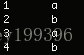# Lua — using remove to delete table data

in Lua, everything is table, all the data, functions are stored in table, but when we use table, how to clean up the table table data.
first see a function:
table.remove(table[,pos]) : delete the element on pos position, the latter element will move forward one, and then the deleted index will move forward, resulting in the deleted data is not what you want. When pos is not filled in, the data at the end of the table is deleted.
see the following code:

``````local array = {"a","a","a","b","a","a","b"}
for i,v in ipairs(array) do
if v == "a" then
table.remove(array, i)
end
end
for i,v in ipairs(array) do
print(i,v)
end``````

output result:we wanted to delete all “a” data in the table, but the output result did not delete all “a” data. So we want to continuously delete the table data can not be used in this way, here is the correct way to delete.

``````local array = {"a","a","a","b","a","a","b"}
for i=#array,1,-1 do
if array[i] == "a" then
table.remove(array, i)
end
end
for i,v in ipairs(array) do
print(i,v)
end``````

so you get the correct deletion.
so we can encapsulate a function

``````
-- 删除table表中符合conditionFunc的数据
-- @param tb 要删除数据的table
-- @param conditionFunc 符合要删除的数据的条件函数
local function table.removeTableData(tb, conditionFunc)
-- body
if tb ~= nil and next(tb) ~= nil then
-- todo
for i = #tb, 1, -1 do
if conditionFunc(tb[i]) then
-- todo
table.remove(tb, i)
end
end
end
end

-- 删除数据是否符合条件
-- @param data 要删除的数据
-- @return 返回是否符合删除data条件
local function removeCondition(data)
-- body
-- TODO data 符合删除的条件
return true
end``````

we just need to write TODO and pass in the table.